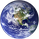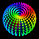552 views
552
This is Covariance on Covariance. It shows you how much a given covariance period has deviated from it mean over another defined period. Because it is a time series, It can allow you to spot changes in how covariance changes. You can apply trend lines , Fibonacci retracements, etc. This is also volume weighting covariance.

This is not a directional indicator nor is moving covariance. This is used for forecasting volatility . This must be used in conjunction with moving covariance.
//Moving Co-Covariance
study(title="Co-Covariance", shorttitle="MCCV", overlay=false)
src = vwap, len = input(30, minval=1, title="covariance length"), len2 = input(7, minval=1, title="Co-covariance look back length")
mean = vwma(src, len)
stdev = stdev(src, len)
covariance = (stdev/mean)
covariancemean = vwma(covariance, len2)
cstdev = stdev(covariance, len2)
cocovariance = (cstdev/covariancemean)
plot(cocovariance, title="covariance on covariance", style = line, color = aqua)I would like to apologize, when naming this indicator I mixed up my terminology. This is coefficent of variation which shows the % a price deviates from its mean. Also known as unitized risk. In this case unitized risk of coefficent of variationIn this indicator I forgot to multiply the result by 100. 0.0928 would be 9.28% etc. A small adjustment to the code will alleviate this. Just multiply cocovariance by 100, but if you like to read it as decimal points then this shouldn't matter to you.interesting, so based on this info ... we can expect a downward motion soon?